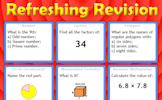# Multiplicative reasoning

### Term 2 starting in week 1 :: Estimated time: 2 weeks

• Use scale factors (review)
• Understand direct proportion
• Calculate with pressure and density
• Understand inverse proportion
• Ratio problems (review)

For higher-attaining pupils:

• Construct complex direct proportion equations
• Construct inverse proportion equations

This page should remember your ticks from one visit to the next for a period of time. It does this by using Local Storage so the information is saved only on the computer you are working on right now.

## Lesson Starters

Here are some suggestions for whole-class, projectable resources which can be used at the beginnings of each lesson in this block.

### 1st Lesson#### Plant More Trees

If six girls can plant 90 trees in a day. How many trees can ten girls plant in a day? The unitary method.

### 2nd Lesson#### Happy New Year

Work out the date for various given amounts of time after the beginning of the year.

### 3rd Lesson#### Giraffe

The height of this giraffe is three and a half metres plus half of its height. How tall is the giraffe?

### 4th Lesson#### Light Shopping

A lamp and a bulb together cost 32 pounds. The lamp costs 30 pounds more than the bulb. How much does the bulb cost?

### 5th Lesson#### Missing Pound

A puzzle about a restaurant bill. Exactly where did the missing pound go?

### 6th Lesson#### Refreshing Revision

It is called Refreshing Revision because every time you refresh the page you get different revision questions.

Some of the Starters above are to reinforce concepts learnt, others are to introduce new ideas while others are on unrelated topics designed for retrieval practice or and opportunity to develop problem-solving skills.

White Rose ResourcesEnd of block assessments provide a quick progress check at the end of each block of learning to make sure students have understood the content covered. This Scheme of Learning was produced by White Rose Maths and is used here with permission granted on 30th June 2021.For All: